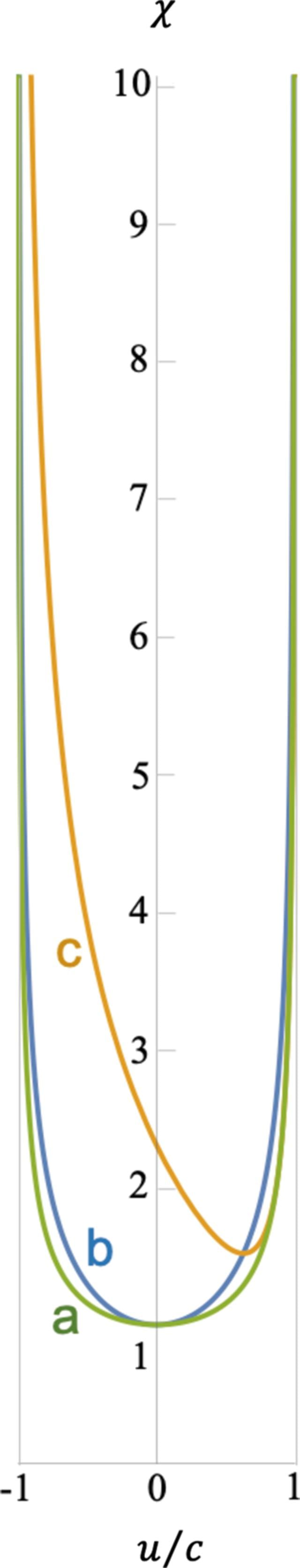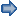disable zoom     view article Figure 3 Plots of the renormalization factorfrom equation (8)as a function offor (a) v = u,, (b) v = 0,, (c) v = 0.9c. The light lines correspond to the vertical asymptotes at. See the Mathematica script in the Mathematica notebook in the supporting information to generate this plot.FOUNDATIONSADVANCES
ISSN: 2053-2733
Volume 77| Part 4| July 2021| Pages 242-256